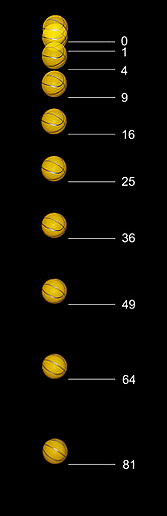# Instantaneous Velocity: How to Find itWhat is the velocity of a falling ball at a particular point in time?The instantaneous velocity is just the velocity of an object at a specific point in time. With calculus, you can find it when given the displacement function, a function which tells you distance moved.

## Instantaneous Velocity Formula

The formula is expressed algebraically as:Where:

• v = Instantaneous velocity (m/s)
• Δx = Vector change in position (m)
• Δt = Change in time (s)
• dr/dt = Derivative of vector position with respect to time (m/s)

## Example 1

Example question: A ball rolls along a sidewalk toward a gutter. The position of the ball on the sidewalk is:
x(t) = 0.000015t5– 0.004t3 + 0.4t.
Position x is in meters while time t is in seconds.  What is the ball’s instantaneous velocity at t = 10.0 s?

Step 1: Plug the above into the formula:

v = d/dt x(t)

v = d/dt (0.000015t5– 0.004t3 + 0.4t)

Step 2: Solve for d/dt:

1. v = 0.000015 d/dt t5 – 0.004 d/dt t3 + 0.4 d/dt t
2. v = 0.000015(5t4) – 0.004(3t) + 4 (dt/dt)
3. v = 0.000075t4 – 0.012t2 + 0.4(1)
4. v = 0.000075t4– 0.012t2 + 0.4

Step 3: Insert time (in this example, that’s 10.0 s) in place of t, then simplify:

1. v = 0.000075(10.0)4 – 0.012(10.0)2 + 0.4 m/s
2. v = 0.75 – 1.20 + 0.4 m/s
3. v = -0.05 m/s

The ball’s instantaneous velocity at 10.0s is 0.05 m/s second in the -x direction.

## Example 2

Example question: Given a displacement function, set the equation up to solve for velocity. For the example we will use a simple problem to illustrate the concept.
x(t) = t3 + t2 + t + 1
v(t) = dx/dt = d/dt (t3 + t2 + t + 1)

Step 1: Use the Power Rule and rule for derivative of constants to solve for the derivative of the displacement function. More complicated functions might necessitate a better knowledge of the rules of differentiation, but these rules work for the example.
v(t) = dx/dt = d/dt (t3 + t2 + t +1) = 3t2 + 2t + 1

Step 2: Now that you have the formula for velocity, you can find the instantaneous velocity at any point. For the example, we will find the instantaneous velocity at 0, which is also referred to as the initial velocity.
v(0) = 3*(02) + 2*(0) + 1 = 1
This indicates the instantaneous velocity at 0 is 1. If you need to find the instantaneous velocity at multiple points, you can simply substitute for t as necessary. For instance, if you needed to find the velocity at 5 as well as 0, just solve for v(5)
v(5) = 3*(52) + 2(5) + 1 = 3 *25 + 10 + 1 = 86

Tip: When performing the operation in typical physics applications, you will need to make sure to include the units of measurement. Velocity is given in units of distance per time.
An interesting note is that at a change in time of 0, there is technically no velocity of the object as motion requires time to pass in order to occur. This is why we take the limit as the change in time approaches 0, instead of simply considering it at 0.

## Instantaneous Velocity vs. Average Velocity

Instantaneous velocity may not be the same as the average velocity.  To illustrate the difference let’s take a typical commute to work.  When sitting in the car in your driveway in the morning the car’s velocity is zero.  Once the car pulls out into the street and you begin your drive the car’s velocity is no longer zero.  The car’s instantaneous velocity will become zero again, when you stop at a light or arrive at work, for example.  However, the average velocity for the trip won’t be zero, even though the instantaneous velocity was zero for portions of the commute.

CITE THIS AS:
Stephanie Glen. "Instantaneous Velocity: How to Find it" From StatisticsHowTo.com: Elementary Statistics for the rest of us! https://www.statisticshowto.com/calculus-problem-solving/instantaneous-velocity/